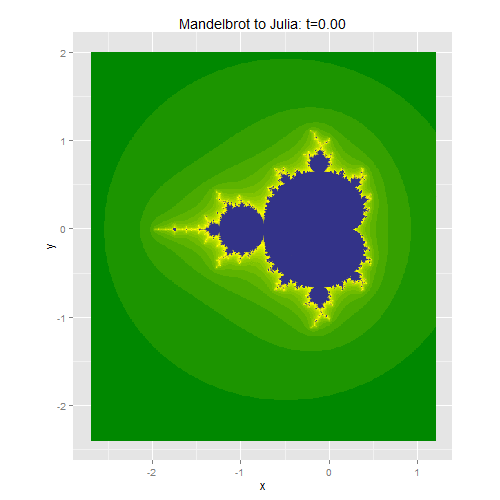Home > Misc

# Fractal morphing

To test if a point $$p \in \mathbb{C}$$ is in the Mandelbrot set, we start with $$z_0 = 0$$ and see if the sequence defined by $$z_{n+1} = z_n^2 + p$$ remains bounded.

The definition of the Julia set includes another constant parameter $$c$$, which below I set to $$0.4 + 0.6i$$. We start with $$z_0 = p$$ and see if the sequence defined by $$z_{n+1} = z_n^2 + c$$ remains bounded. (Strictly speaking, the Julia set is the boundary between the points which diverge to infinity and those which remain bounded. That subtlety isn't important when trying to make pretty pictures. The Julia set is also defined on more general functions than $$z^2 + c$$.)

The similarity between the two definitions makes it natural to try morphing between them, introducing a parameter $$t$$ such that we get the Mandelbrot set at $$t=0$$ and the Julia set at $$t=1$$. For each point $$p$$, we set $$z_0 = tp$$ and iterate $$z_{n+1} = z_n^2 + p + t(c - p)$$.

The resulting morph:R code follows. It is quite slow.

library(ggplot2)

x <- seq(-2.7, 1.2, length.out=500)
y <- seq(-2.4, 2, length.out=500)
grid <- expand.grid(x=x, y=y)
grid$p <- complex(real=grid$x, imag=grid$y) # Julia fractal constant: c <- complex(real=0.4, imag=0.6) num_iter <- 50 t_vec <- seq(0, 1, length.out=51) t_ct <- 1 for (t in t_vec) { print(t) grid$clr <- num_iter
grid$z <- t*grid$p
not_diverged <- which(abs(grid$z) <= 4) for (ct in 1:num_iter) { grid$z <- grid$z * grid$z + grid$p + t*(c - grid$p)
diverged <- which(abs(grid$z) > 4) new_diverged <- intersect(not_diverged, diverged) grid$clr[new_diverged] <- ct

not_diverged <- which(abs(grid$z) <= 4) } # I think this tweak makes the pictures look nicer: grid$clr <- sqrt(grid\$clr)

colour_vec <- c("#008800", "#FFFF00", "#333388")
title_str <- sprintf("Mandelbrot to Julia: t=%2.2f", t)

fractal_plot <- ggplot() +
geom_raster(data=grid, aes(x=x, y=y, fill=clr)) +
guides(fill=F) + coord_equal() +
}